If you have a few years of experience in the Java ecosystem, and you’d like to share that with the community, have a look at our Contribution Guidelines.

### Get started with Spring 5 and Spring Boot 2, through the Learn Spring course:

>> CHECK OUT THE COURSE

## 1. Introduction

In this article, we'll see how to find the kth-smallest element in the union of two sorted arrays.

First, we'll define the exact problem. Second, we'll see two inefficient but straightforward solutions. Third, we'll look at an efficient solution based on a binary search on the two arrays. Finally, we'll look at some tests to verify that our algorithm works.

We'll also see Java code snippets for all parts of the algorithm. For simplicity, our implementation will only operate on integers. However, the described algorithm works with all data types that are comparable and could even be implemented using Generics.

## 2. What Is the Kth Smallest Element in the Union of Two Sorted Arrays?

### 2.1. The Kth Smallest Element

To find the kth-smallest element, also called the kth-order statistic, in an array, we typically use a selection algorithm. However, these algorithms operate on a single, unsorted array, whereas in this article, we want to find the kth smallest element in two sorted arrays.

Before we see several solutions to the problem, let's exactly define what we want to achieve. For that, let's jump right into an example.

We are given two sorted arrays (a and b), which do not necessarily need to have an equal number of elements: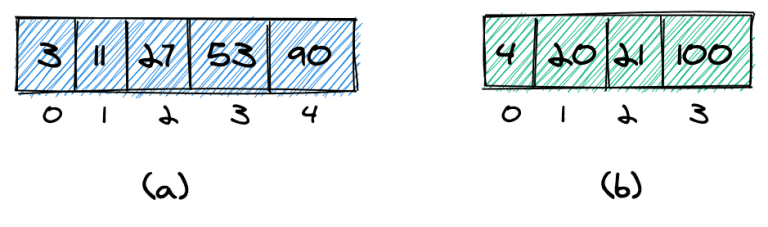In these two arrays, we want to find the kth smallest element. More specifically, we want to find the kth smallest element in the combined and sorted array: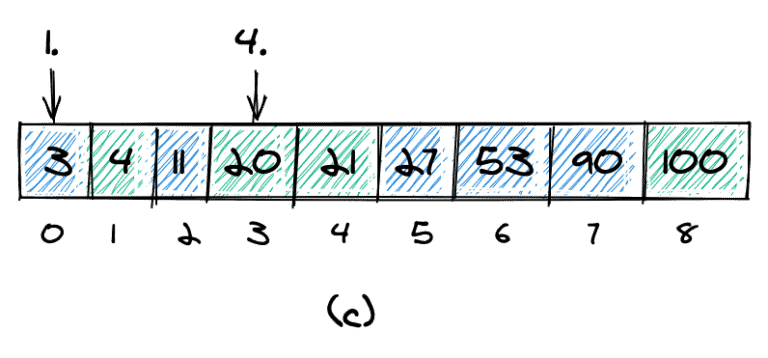The combined and sorted array for our example is shown in (c). The 1st smallest element is 3, and the 4th smallest element is 20.

### 2.2. Duplicate Values

We'll also need to define how to handle duplicate values. An element could occur more than once in one of the arrays (element 3 in array a) and also occur again in the second array (b).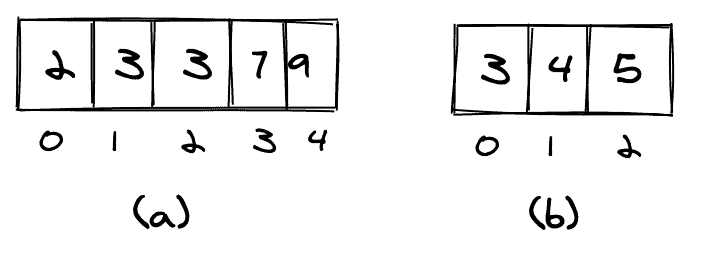If we count duplicates only once, we'll count as shown in (c). If we count all occurrences of an element, we'll count as shown in (d).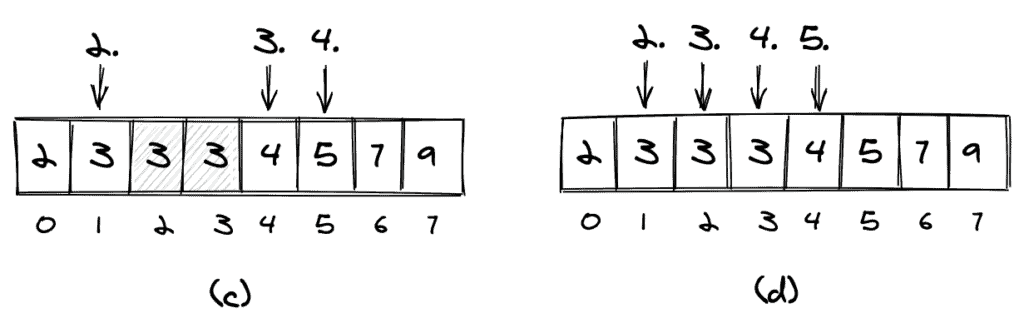In the remaining part of this article, we'll count duplicates as shown in (d), thus counting them as if they were distinct elements.

## 3. Two Simple but Less Efficient Approaches

### 3.1. Join and Then Sort the Two Arrays

The simplest way to find the kth smallest element is to join the arrays, sort them, and return the kth element of the resulting array:

``````int getKthElementSorted(int[] list1, int[] list2, int k) {

int length1 = list1.length, length2 = list2.length;
int[] combinedArray = new int[length1 + length2];
System.arraycopy(list1, 0, combinedArray, 0, list1.length);
System.arraycopy(list2, 0, combinedArray, list1.length, list2.length);
Arrays.sort(combinedArray);

return combinedArray[k-1];
}``````

With n being the length of the first array and m the length of the second array, we get the combined length c = n + m.

Since the complexity for the sort is O(c log c), the overall complexity of this approach is O(n log n).

A disadvantage of this approach is that we need to create a copy of the array, which results in more space needed.

### 3.2. Merge the Two Arrays

Similar to one single step of the Merge Sort sorting algorithm, we can merge the two arrays and then directly retrieve the kth element.

The basic idea of the merge algorithm is to start with two pointers, which point to the first elements of the first and second arrays (a).

We then compare the two elements (3 and 4) at the pointers, add the smaller one (3) to the result, and move that pointer one position forward (b). Again, we compare the elements at the pointers and add the smaller one (4) to the result.

We continue in the same way until all elements are added to the resulting array. If one of the input arrays does not have more elements, we simply copy all of the remaining elements of the other input array to the result array.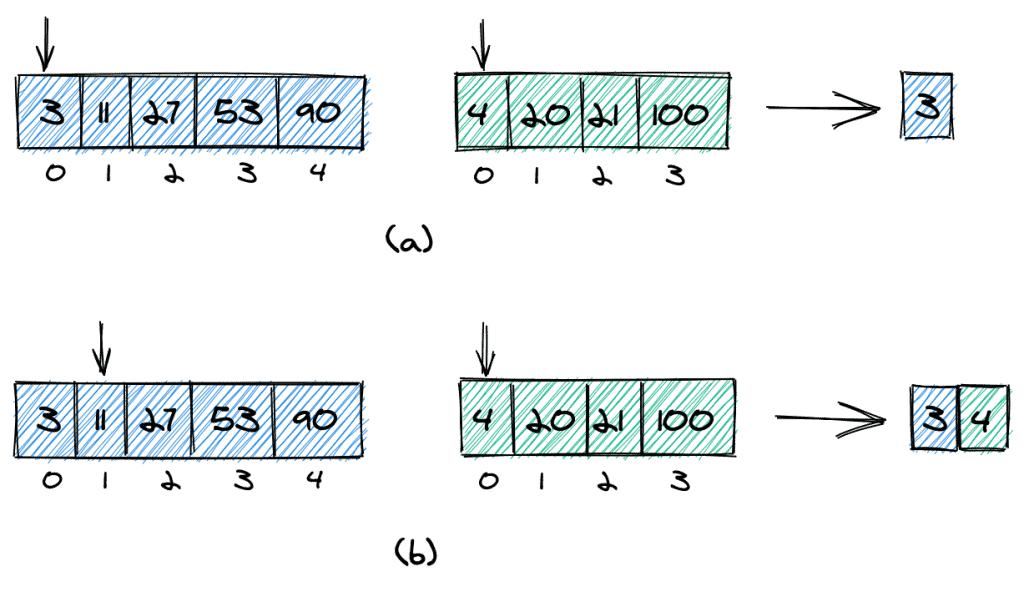We can improve the performance if we don't copy the full arrays, but stop when the resulting array has k elements. We don't even need to create an additional array for the combined array but can operate on the original arrays only.

Here's an implementation in Java:

``````public static int getKthElementMerge(int[] list1, int[] list2, int k) {

int i1 = 0, i2 = 0;

while(i1 < list1.length && i2 < list2.length && (i1 + i2) < k) {
if(list1[i1] < list2[i2]) {
i1++;
} else {
i2++;
}
}

if((i1 + i2) < k) {
return i1 < list1.length ? list1[k - i2 - 1] : list2[k - i1 - 1];
} else if(i1 > 0 && i2 > 0) {
return Math.max(list1[i1-1], list2[i2-1]);
} else {
return i1 == 0 ? list2[i2-1] : list1[i1-1];
}
}``````

It's straightforward to understand the time-complexity of this algorithm is O(k). An advantage of this algorithm is that it can be easily adapted to consider duplicate elements only once.

## 4. A Binary Search Over Both Arrays

Can we do better than O(k)? The answer is that we can. The basic idea is to do a binary search algorithm over the two arrays.

For this to work, we need a data structure that provides constant-time read access to all its elements. In Java, that could be an array or an ArrayList.

Let's define the skeleton for the method we are going to implement:

``````int findKthElement(int k, int[] list1, int[] list2)
throws NoSuchElementException, IllegalArgumentException {

// check input (see below)

// handle special cases (see below)

// binary search (see below)
}``````

Here, we pass k and the two arrays as arguments. First, we'll validate the input; second, we handle some special cases and then do the binary search. In the next three sections, we'll look at these three steps in reverse order, so first, we'll see the binary search, second, the special cases, and finally, the parameter validation.

### 4.1. The Binary Search

The standard binary search, where we are looking for a specific element, has two possible outcomes: either we find the element we're looking for and the search is successful, or we don't find it and the search is unsuccessful. This is different in our case, where we want to find the kth smallest element. Here, we always have a result.

Let's look at how to implement that.

#### 4.1.1. Finding the Correct Number of Elements From Both Arrays

We start our search with a certain number of elements from the first array. Let's call that number nElementsList1. As we need k elements in total, the number nElementsList1 is:

``````int nElementsList2 = k - nElementsList1;
``````

As an example, let's say k = 8. We start with four elements from the first array and four elements from the second array (a).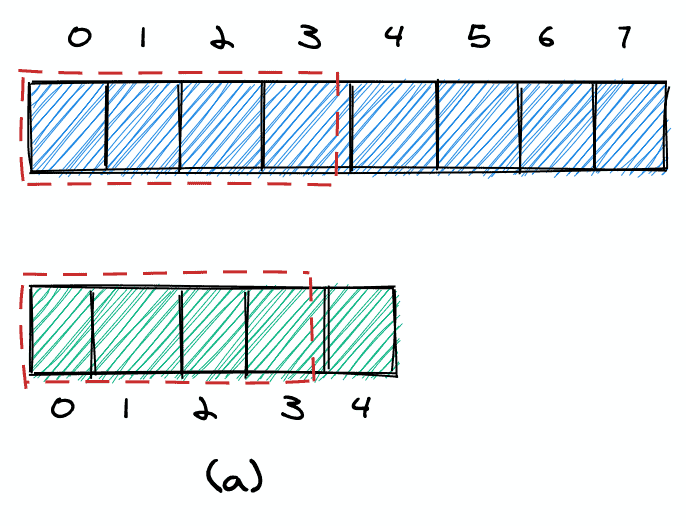If the 4th element in the first array is bigger than the 4th element in the second array, we know that we took too many elements from the first array and can decrease nElementsList1 (b). Otherwise, we know that we took too few elements and can increase nElementsList1 (b').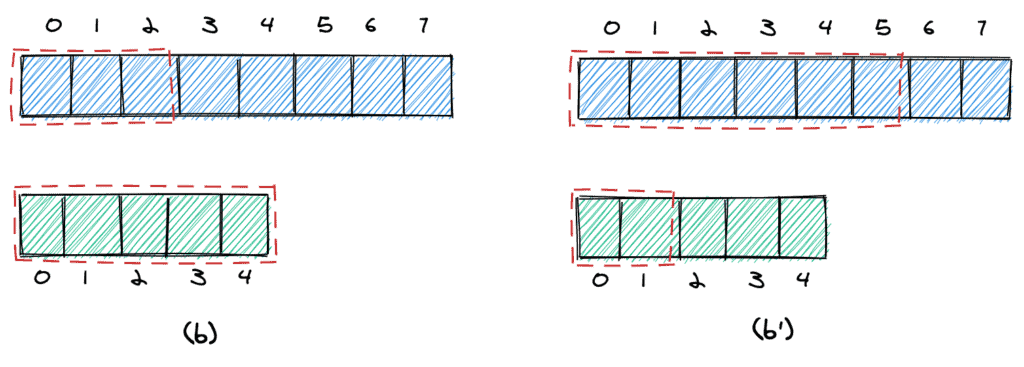We continue until we have reached the stopping criteria. Before we look at what that is, let's look at the code for what we've described so far:

``````int right = k;
int left = = 0;
do {
nElementsList1 = ((left + right) / 2) + 1;
nElementsList2 = k - nElementsList1;

if(nElementsList2 > 0) {
if (list1[nElementsList1 - 1] > list2[nElementsList2 - 1]) {
right = nElementsList1 - 2;
} else {
left = nElementsList1;
}
}
} while(!kthSmallesElementFound(list1, list2, nElementsList1, nElementsList2));``````

#### 4.1.2. Stopping Criteria

We can stop in two cases. First, we can stop if the maximum element we take from the first array is equal to the maximum element we take from the second (c). In this case, we can simply return that element.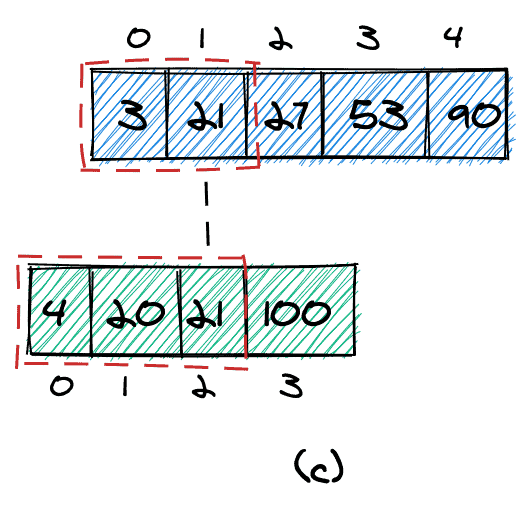Second, we can stop if the following two conditions are met (d):

• The largest element to take from the first array is smaller than the smallest element we do not take from the second array (11 < 100).
• The largest element to take from the second array is smaller than the smallest element we do not take from the first array (21 < 27).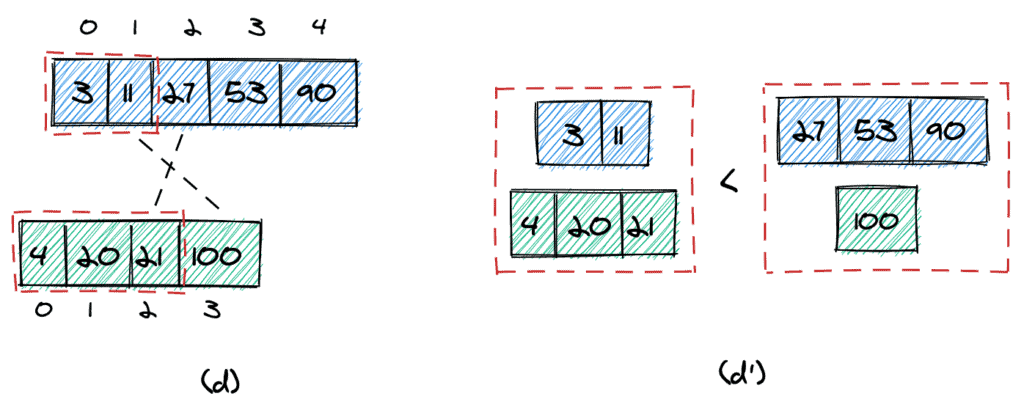It's easy to visualize (d') why that condition works: all elements we take from the two arrays are surely smaller than any other element in the two arrays.

Here's the code for the stopping criteria:

``````private static boolean foundCorrectNumberOfElementsInBothLists(int[] list1, int[] list2, int nElementsList1, int nElementsList2) {

// we do not take any element from the second list
if(nElementsList2 < 1) {
return true;
}

if(list1[nElementsList1-1] == list2[nElementsList2-1]) {
return true;
}

if(nElementsList1 == list1.length) {
return list1[nElementsList1-1] <= list2[nElementsList2];
}

if(nElementsList2 == list2.length) {
return list2[nElementsList2-1] <= list1[nElementsList1];
}

return list1[nElementsList1-1] <= list2[nElementsList2] && list2[nElementsList2-1] <= list1[nElementsList1];
}``````

#### 4.1.3. The Return Value

Finally, we need to return the correct value. Here, we have three possible cases:

• We take no elements from the second array, thus the target value is in the first array (e)
• The target value is in the first array (e')
• The target value is in the second array (e”)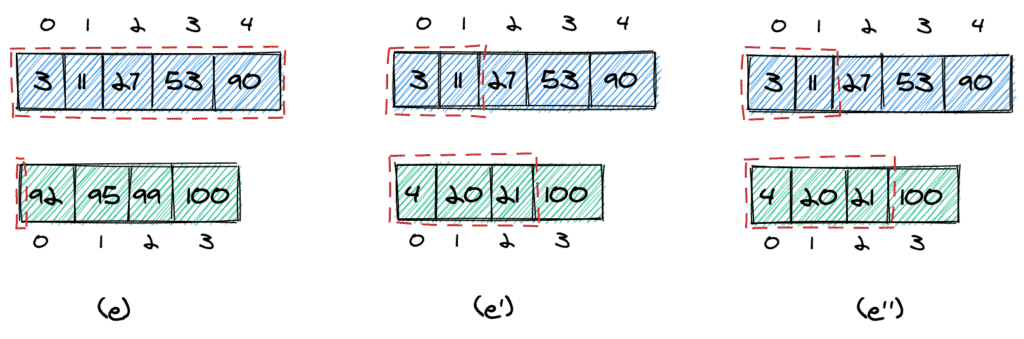Let's see this in code:

``return nElementsList2 == 0 ? list1[nElementsList1-1] : max(list1[nElementsList1-1], list2[nElementsList2-1]);``

Note that we do not need to handle the case where we don't take any element from the first array — we'll exclude that case in the handling of special cases later.

### 4.2. Initial Values for the Left and Right Borders

Until now, we initialized the right and left border for the first array with k and 0:

``````int right = k;
int left = 0;``````

However, depending on the value of k, we need to adapt these borders.

First, if k exceeds the length of the first array, we need to take the last element as the right border. The reason for this is quite straightforward, as we cannot take more elements from the array than there are.

Second, if k is bigger than the number of elements in the second array, we know for sure that we need to take at least (k – length(list2)) from the first array. As an example, let's say k = 7. As the second array only has four elements, we know that we need to take at least 3 elements from the first array, so we can set L to 2: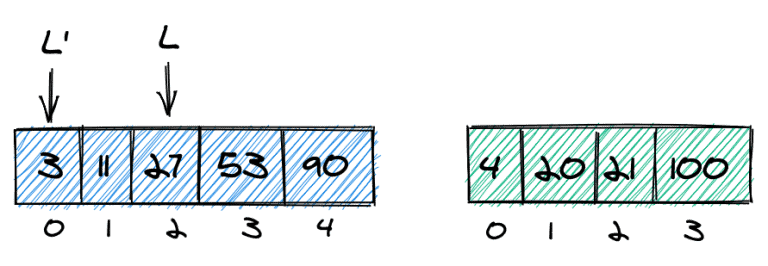Here's the code for the adapted left and right borders:

``````// correct left boundary if k is bigger than the size of list2
int left = k < list2.length ? 0 : k - list2.length - 1;

// the inital right boundary cannot exceed the list1
int right = min(k-1, list1.length - 1);``````

### 4.3. Handling of Special Cases

Before we do the actual binary search, we can handle a few special cases to make the algorithm slightly less complicated and avoid exceptions. Here's the code with explanations in the comments:

``````// we are looking for the minimum value
if(k == 1) {
return min(list1, list2);
}

// we are looking for the maximum value
if(list1.length + list2.length == k) {
return max(list1[list1.length-1], list2[list2.length-1]);
}

// swap lists if needed to make sure we take at least one element from list1
if(k <= list2.length && list2[k-1] < list1) {
int[] list1_ = list1;
list1 = list2;
list2 = list1_;
}``````

### 4.4. Input Validation

Let's look at the input validation first. To prevent the algorithm from failing and throwing, for example, a NullPointerException or ArrayIndexOutOfBoundsException, we want to make sure that the three parameters meet the following conditions:

• Both arrays must not be null and have at least one element
• k must be >= 0 and cannot be bigger than the length of the two arrays together

Here's our validation in code:

``````void checkInput(int k, int[] list1, int[] list2) throws NoSuchElementException, IllegalArgumentException {

if(list1 == null || list2 == null || k < 1) {
throw new IllegalArgumentException();
}

if(list1.length == 0 || list2.length == 0) {
throw new IllegalArgumentException();
}

if(k > list1.length + list2.length) {
throw new NoSuchElementException();
}
}``````

### 4.5. Full Code

Here's the full code of the algorithm we've just described:

``````public static int findKthElement(int k, int[] list1, int[] list2) throws NoSuchElementException, IllegalArgumentException {

checkInput(k, list1, list2);

// we are looking for the minimum value
if(k == 1) {
return min(list1, list2);
}

// we are looking for the maximum value
if(list1.length + list2.length == k) {
return max(list1[list1.length-1], list2[list2.length-1]);
}

// swap lists if needed to make sure we take at least one element from list1
if(k <= list2.length && list2[k-1] < list1) {
int[] list1_ = list1;
list1 = list2;
list2 = list1_;
}

// correct left boundary if k is bigger than the size of list2
int left = k < list2.length ? 0 : k - list2.length - 1;

// the inital right boundary cannot exceed the list1
int right = min(k-1, list1.length - 1);

int nElementsList1, nElementsList2;

// binary search
do {
nElementsList1 = ((left + right) / 2) + 1;
nElementsList2 = k - nElementsList1;

if(nElementsList2 > 0) {
if (list1[nElementsList1 - 1] > list2[nElementsList2 - 1]) {
right = nElementsList1 - 2;
} else {
left = nElementsList1;
}
}
} while(!kthSmallesElementFound(list1, list2, nElementsList1, nElementsList2));

return nElementsList2 == 0 ? list1[nElementsList1-1] : max(list1[nElementsList1-1], list2[nElementsList2-1]);
}

private static boolean foundCorrectNumberOfElementsInBothLists(int[] list1, int[] list2, int nElementsList1, int nElementsList2) {

// we do not take any element from the second list
if(nElementsList2 < 1) {
return true;
}

if(list1[nElementsList1-1] == list2[nElementsList2-1]) {
return true;
}

if(nElementsList1 == list1.length) {
return list1[nElementsList1-1] <= list2[nElementsList2];
}

if(nElementsList2 == list2.length) {
return list2[nElementsList2-1] <= list1[nElementsList1];
}

return list1[nElementsList1-1] <= list2[nElementsList2] && list2[nElementsList2-1] <= list1[nElementsList1];
}``````

## 5. Testing the Algorithm

In our GitHub repository, there are many test cases that cover a lot of possible input arrays and also many corner cases.

Here, we only point out one of the tests, which tests not against static input arrays but compares the result of our double binary search algorithm to the result of the simple join-and-sort algorithm. The input consists of two randomized arrays:

``````int[] sortedRandomIntArrayOfLength(int length) {
int[] intArray = new Random().ints(length).toArray();
Arrays.sort(intArray);
return intArray;
}``````

The following method performs one single test:

``````private void random() {

Random random = new Random();
int length1 = (Math.abs(random.nextInt())) % 1000 + 1;
int length2 = (Math.abs(random.nextInt())) % 1000 + 1;

int[] list1 = sortedRandomIntArrayOfLength(length1);
int[] list2 = sortedRandomIntArrayOfLength(length2);

int k = (Math.abs(random.nextInt()) + 1) % (length1 + length2);

int result = findKthElement(k, list1, list2);
int result2 = getKthElementSorted(list1, list2, k);
int result3 = getKthElementMerge(list1, list2, k);

assertEquals(result2, result);
assertEquals(result2, result3);
}``````

And we can call the above method to run a large number of tests like that:

``````@Test
void randomTests() {
IntStream.range(1, 100000).forEach(i -> random());
}``````

## 6. Conclusion

In this article, we saw several ways of how to find the kth smallest element in the union of two sorted arrays. First, we saw a simple and straightforward O(n log n) algorithm, then a version with complexity O(n), and last, an algorithm that runs in O(log n).

The last algorithm we saw is a nice theoretical exercise; however, for most practical purposes, we should consider using one of the first two algorithms, which are much simpler than the binary search over two arrays. Of course, if performance is an issue, a binary search could be a solution.